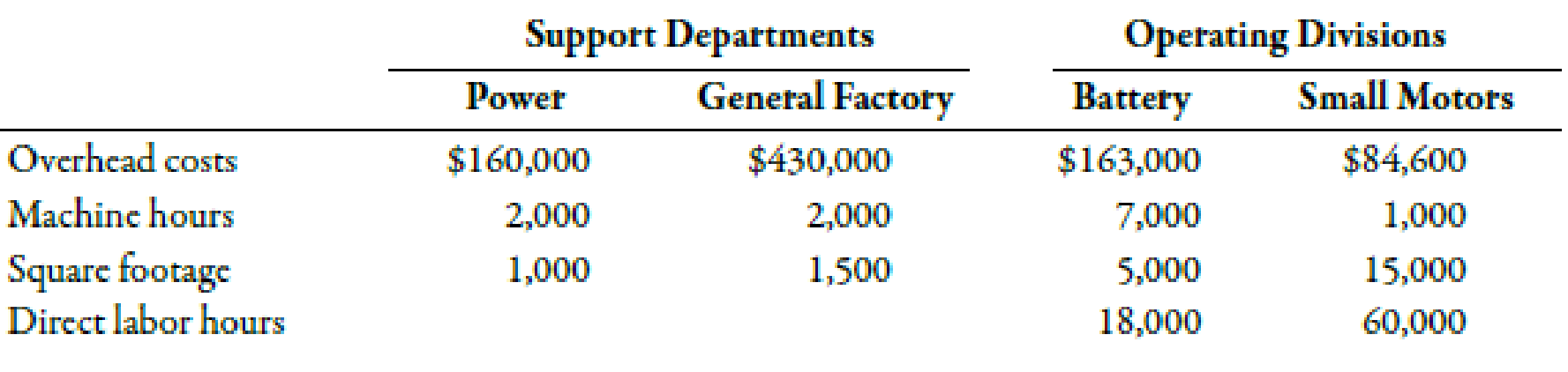Chapter 4, Problem 51E### Managerial Accounting: The Corners...

7th Edition
Maryanne M. Mowen + 2 others
ISBN: 9781337115773

#### Solutions

Chapter
Section### Managerial Accounting: The Corners...

7th Edition
Maryanne M. Mowen + 2 others
ISBN: 9781337115773
Textbook Problem
18 views

# (Appendix 4B) Direct Method of Support Department Cost AllocationStevenson Company is divided into two operating divisions: Battery and Small Motors. The company allocates power and general factory costs to each operating division using the direct method. Power costs are allocated on the basis of the number of machine hours and general factory costs on the basis of square footage. Support department cost allocations using the direct method are based on the following data:Required: 1. Calculate the allocation ratios for Power and General Factory. (Note: Carry these calculations out to four decimal places.) 2. Allocate the support service costs to the operating divisions. (Note: Round all amounts to the nearest dollar.) 3. Assume divisional overhead rates are based on direct labor hours. Calculate the overhead rate for the Battery Division and for the Small Motors Division. (Note: Round overhead rates to the nearest cent.)

1.

To determine

Compute allocation ratios for power and general factory.

Explanation

Direct Method:

Direct method implies that the unit cost must include all the factory costs. It states that the cost of support departments should not be added in the unit cost if it is not included in producing departments’ cost because they do not play any role in selling the unit or product.

Use the following formula to calculate allocation ratios for power on the basis of number machine hours:

Battery:

Battery=NumberofmachinehoursinbatteryTotalmachinehoursinoperatingdepartments

Substitute 7,000mhrsfor number of machine hours in battery and 8,000mhrsfor total machine hours in operating department in the above formula.

Battery=7,000mhrs8,000mhrs=0.8750

Therefore, the assignment ratio for cutting department is 0.8750.

Small motors:

Smallmotors=NumberofmachinehoursinsmallmotorsTotalmachinehoursinoperatingdepartments

Substitute 1,000 mhrs for number of employees in cutting and 8,000 mhrs for total employees in producing department in the above formula.

Smallmotors=1,000mhrs8,000mhrs=0

2.

To determine

Apportion support service costs to the operating divisions:

3.

To determine

Find out the overhead rate of battery and small motors divisions.

### Still sussing out bartleby?

Check out a sample textbook solution.

See a sample solution

#### The Solution to Your Study Problems

Bartleby provides explanations to thousands of textbook problems written by our experts, many with advanced degrees!

Get Started

#### Find more solutions based on key concepts